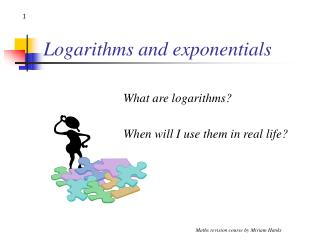DownloadDownload PresentationLogarithms and exponentials

# Logarithms and exponentials

Download Presentation## Logarithms and exponentials

- - - - - - - - - - - - - - - - - - - - - - - - - - - E N D - - - - - - - - - - - - - - - - - - - - - - - - - - -
##### Presentation Transcript

1. Logarithms and exponentials What are logarithms? When will I use them in real life? Maths revision course by Miriam Hanks

2. Logarithms and exponentials • Uses of logarithms in real life: • Earthquakes (Richter scale) • Sound (Decibel scale) • Chemistry: “Half-life” • Biology: Growth and decay in nature Maths revision course by Miriam Hanks

3. Logarithms and exponentials Imagine you are studying acoustics, and want to be able to plot a graph so that the sound of a whisper and the roar of a jet engine both fit on the scale. Maths revision course by Miriam Hanks

4. Logarithms and exponentials The problem is that a jet is about 10100 times louder than a whisper So why not just use a scale so that they both fit in? Because then we wouldn’t have enough space to clearly see the whisper separately to talking at normal volume. jet whisper 0 Maths revision course by Miriam Hanks

5. Logarithms and exponentials So instead, we use a scale where the units represent powers of 10 This graph would only need to go up 120 squares to get to 10120, which is how loud a jet engine is. What is the advantage of this method? 105 104 103 102 10 0 Maths revision course by Miriam Hanks

6. Logarithms and exponentials • The advantage is that the “small” end of the graph is not as squashed, so you can still see a whisper and other sounds in that range. It only squashes the “big” end of the graph! Maths revision course by Miriam Hanks

7. 5 4 3 2 1 0 Logarithms and Exponentials Now, scientists can’t be bothered to write the “10” each time, so they just write the power. This is now the Decibel scale. Every time you go up 1 unit, you multiply by 10. 105 104 103 102 10 0 Maths revision course by Miriam Hanks

8. Logarithms and exponentials The Richter scale for earthquakes is also logarithmic. The quake that caused the Tsunami tidal wave in Japan in 2011 was 8.9 on the Richter scale. The one in Christchurch, NZ in 2010 was 6.3. How many times bigger is that? 108.9  106.3400 times bigger Maths revision course by Miriam Hanks

9. Logarithms You need to know what log28 means: It means “what power of 2 makes 8?” So, the answer is 3. Maths revision course by Miriam Hanks

10. Logarithms You need to remember and use the 4 log laws: logx a + logx b = logx ab logx a – logx b = logx (a/b) logx an = nlogx a logx a = b means xb = a and vice versa Maths revision course by Miriam Hanks

11. Natural logarithms e is the number 2.71.... It is also called Euler’s number loge x is also written as ln x Maths revision course by Miriam Hanks

12. Solving equations with logs To solve an equation with an unknown power, you will need to use logs. eg 3x+1 = 5 log 3x+1 = log 5 (x + 1)log3 = log5 x + 1 = log5 / log3 x = log5/log3 – 1 = 0.465 (to 3dps) Maths revision course by Miriam Hanks